The GPS and the Constant Velocity of Light
Paul Marmet

( Last checked 2018/01/15 - The estate of Paul Marmet )
Return to:    List of Papers on Web Sites
Abstract
When the velocity of light is measured with the Global Positioning System (GPS), we find that it is (c-v) or (c+v), in which v is the rotation velocity of the Earth where the cities are located.  We know that the Lorentz transformations and special relativity are unable to provide a realistic physical explanation of the behavior of matter and light. We show here that all these phenomena can be explained using Newton's physics and mass-energy conservation, without space contraction or time dilation. We have seen previously  that the principle of mass-energy conservation requires that clocks run at a slower rate in a moving frame, and physical bodies become longer because of the increase of the Bohr radius. These results allow us to answer the question: With respect to what, does light travel? For example, when we move away at velocity v, from a source emitting light at velocity c, the relative motion of the radiation is observed from the Doppler shift. How can we explain logically that these photons "appear" to reach us at velocity c and not (c-v)? The conventional explanation relies on special relativity, but it implies an esoteric space-time distortion.
This paper gives a physical explanation how the velocity of light is really (c-v) with respect to the observer, even if the observer's tools always measure a velocity represented by the number c. We explain how this problem is crucial in the Global Positioning System (GPS) and in clocks synchronization.  The Lorentz' transformations become quite useless. This apparent constant velocity of light with respect to a moving frame is the most fascinating illusion in science.

1 - Introduction
Many experiments, like the Michelson-Morley and Sagnac experiments and others, are testing the fundamental nature of light. It is conflicting to observe that the velocity of photons is measured as a constant, when the observer moves away from that light source. Since all other particles are measured with additive velocities (V-v) or (V+v) with respect to a moving frame, why can photons not obey that same rule? Since Newton's mechanics has shown that all relative velocities produce a Doppler frequency shift, we must expect that some special phenomena prevent us from detecting the real change of relative velocity. It is quite incorrect to believe that this phenomenon cannot be explained using physical reality and Newton physics.  As required by the principle of mass-energy conservation , the atoms (nucleus and electrons) forming the local standard reference meter and the moving clock have acquired some extra mass due to the materialization of kinetic energy. Quantum mechanics shows  that this increase of energy changes the de Broglie electron wavelength and consequently, the Bohr radius and the clock rate. It is surprising to find new hypotheses like space-time distortion, and even more, the suggestion of "new logic" to explain these observations, while it is not taken into account that the rate of the moving clock is naturally modified due to the increase of mass (following the absorption of kinetic energy). The simple application of the principle of mass-energy conservation explains naturally all these experiments.
We must recall that an empirical equation used to predict the outcome of a physical system is not an explanation. When there is no physics underneath these mathematical equations, they give empirical predictions of what will happen to the system. Mathematical equations generally deal with symbols, but they never explain "why". A real explanation must answer the question of causality, which is asked by why? An equation is never the "cause" of a phenomenon.

2 - Switching between Frames of Reference
Let us consider the frame of reference of a small stars cluster, with stars having all the same velocity, as illustrated on figure 1. One of those stars is our Sun, which is surrounded by the Earth moving around it. In this star frame, an observer measures that the photons are emitted at velocity c with respect to the star system. That light (hn, on figure 1) travels toward the Earth, but the Earth moves away at velocity vE with respect to the star system as illustrated on figure 1.Figure 1

That photons are some sort of electromagnetic wave packets, which travel at velocity c with respect to the stars cluster. Consequently, those photons must logically travel at a velocity (c-v) with respect to the Earth that moves at velocity v (see figure 1).  As demonstrated previously , the strict application of the principle of mass-energy conservation leads to the slowing down of clocks and the increase of the Bohr radius, which produces an increase of the physical length of matter. More far-reaching applications have been presented previously , but in the present paper, we need to use solely the increase of length of matter and the slowing down of clocks. Using classical physics with these two natural consequences of mass-energy conservation, this is totally sufficient to explain all the problems related to special relativity. The Lorentz equations become useless. A previous reading of the book  would be extremely helpful, even if the main explanations and relationships are briefly recalled here.
Let us now simplify figure 1. On the right hand side of figure 2, the Earth moving at velocity [v], is now substituted by a train, moving at velocity v with respect to the station frame [s]. An image of the moving train appears on the upper left of figure 2, at a previous time. The physical length of the moving train is established here as Lv, which is the distance between clocks a and ß. Below, we see the train at rest at the station [s] before it started to move. Light emitted from the star system, is now represented by the light emitted at location A on the station frame [s]. On figure 2, the length of the station is the distance Lv between clocks A and B. That same distance is equal to the length of the train "in motion". Of course, the length of the train Ls at rest is shorter before it started to move.Figure 2

As explained previously  the relative length Lv of the train in motion with respect to the train at rest Ls, is:1
The parameter g is equal to 1/(1-(v2/c2))(1/2). Capital letters are used to describe physical lengths. The sub index gives the frame where the physical body is located. To be coherent, the physical length Ls and Lv must be compared with the same standard unit of length in the same frame. We have seen  that when we carry a standard unit of length from a rest frame to a moving frame, that standard length of reference also becomes g times longer. For example, the relationships between the lengths Lv and Ls in equation 1 can be verified experimentally if, at one instant, clocks a and ß on the moving train leaves some marks on the station frame that can be measured with the station meter.
However, since we deal with observers measuring lengths and recording clock displays using their proper units, we need to determine the number of local units in other frames. Of course, when the standard meter used to make measurements is moved to another frame, its physical length is also changed. Therefore when the moving observer determines the length of a moving body, he is now doing it with respect to the local standard meter (which is different). The number "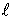" represents the number of times the designated standard units of length have been counted when measuring L. The number of times a (moving) particular length is longer than the standard length located on the station [s], is represented byv[s].
The definition of these indexes is the following. The sub indexes "s" or "v" (ins,v) specify the frame where the body is located when it is measured. The quantity inside the square parenthesis, indicates in which frame [s] or [v], the standard reference unit used for the measurement, is located. We see that the same rod, at different locations, can be designated by four numberss[s],s[v],v[s] orv[v].
We take the example when the observer on the train uses his local meter to measure the length of the moving train. He finds that this numberv[v] is identical to the number of units on the stations[s] before the train started to move, even if it is not the same physical length (Lv>Ls ). However, when the same physical rod, in the same frame (constant Lv) is measured using different standard units [v] or [s], the numbers measured with respect to each standard lengths units [v] or [s] follows  the relationship: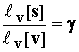2
Since the moving observer uses his local moving standard units, he might believe that the length does not increase when his own velocity increases. He does not realize that his train is physically longer, but this is not measurable, because his local standard meter has increased in the same proportion.  In doing local mathematical calculations, he will normally use the numberv[v] to calculate the length, which is identical to the numbers[s]. In fact equation 2 also implies that the real physical length Lv is equal to g times Ls. In order to apply physics correctly, the moving observer must compensate for the fact that he does not possess the same standard unit of length as when he is located on the station frame. Therefore he must apply a correction due to the change of length of his measuring local standard meter as given in equation 2.
We have seen that, due to mass-energy conservation, it is impossible to switch matter between frames without changing the physical length of the standard measuring meter. For the same reason, it is impossible to switch a standard clock to a new frame without altering its clock rate. At the same time matter passes from a station frame to a moving frame, we have seen (1) that atomic clocks change their rate, because the fundamental particles (electrons, etc.) of the atoms have acquired energy-equivalent mass.  We have seen  that the rate of the moving clocks a and ß (on a moving frame) is g times slower than the rate of clocks A or B located on the rest frame. Consequently, when one local second [v] is measured on the moving train, the moving observer must realize that in fact, a longer time interval has elapsed, because that local moving clock is slow. During exactly the same time interval, the slower clock rate of the moving clock produces a smaller difference of clock display DCDv than the display observed on the rest clock DCDs. The relative Difference of Clock Displays between these frames is given by the relationship: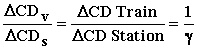3
The train observer must take into account in his calculation that his moving clock is slow. Just as when he was measuring lengths, he knows that the two clocks A and a located on different frames will show a different difference of display (apparent time) during the same real time interval. Using a similar method as length (equation 2), during the same time interval, the moving observer must use equation 3 to compensate for his slow local clock according to the relationship: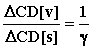4
In this paper, we do not need to consider directly the internal change of mass of electrons and nuclei.  This has been considered previously [1, 2]. Here, there is no change of gravitational potential.  Such a change of gravitational potential has been calculated for the advance of the perihelion of Mercury . Here we deal only with clock rates and physical lengths, which corresponds to special relativity. Consequently, the problem is much simpler. There exists neither space contraction nor time dilation, just a change of length of physical bodies and a change of clock rate.  However, since we deal with velocities, we have seen  previously, that all velocities are represented by identical units (V[s] = V[v]), whether we use the star units or the Earth units, because local lengths and local clock rates vary in the same proportion when switching between frames. Our aim is now to calculate the velocity of light emitted from source A, when measured inside the moving train observer, using the local train clocks and the local moving standard meter.
These calculations imply quantities having a very large variation in size.  In order to avoid lengthy calculations involving different physical phenomena, we will sometimes limit the calculation to the first order (power) of v/c.  Since these calculations are verified by the GPS and the Sagnac effect, we will neglect all higher power of v/c, because they modify the result by a quantity as small as 0.000001 of the relevant calculated Sagnac effect.  Further investigation involving a higher power of v/c will be considered later.

3 - Einstein's Clock Synchronization Technique
On the station frame, an observer calculates the velocity of light, using his proper units [s] and the standard method used by Einstein. A pulse of light is emitted from location A toward B (see figure 2). The station observer measures the velocity of light, calculating the quotient of the length Lv, divided by the difference of local time between light emitted from A and received at B (see figure 2). Since the train is in motion, for the station observer, the distance Lv between A and B is represented byv[s] and nots[s], because the train is really longer when in motion. Measuring the "time interval" means only, that the station observer records the displays shown respectively on both clocks, at the instant light is at location A (CDA) and later B (CDB). This experiment gives c.5
We notice that clocks A, B, a and ß have not been synchronized yet. Let us apply the Einstein's synchronization method to the moving frame. A pulse of light is emitted from location A on the station (see figure 2). Later, at the moment some photons pass through location a, the Clock Display (CDa[v]) on clock a is recorded. Also, when light reaches location ß, the Clock Display on ß (CDß[v]) is recorded. As seen by the train observer, the velocity of light on the moving train is given by the following quotient. --- The distancev[v] (between a and ß) divided by: " the Difference of Clock Display between clock ß, (when light arrives)" minus "the display on clock a (when light passed in a)".
Before calculating correctly the velocity of light on the train, we must synchronize clocks a and ß on the moving frame. As suggested by Einstein, we synchronize clock a with clock ß (inside the moving frame) in the usual way. It is a two-way velocity clock synchronization. The Einstein's synchronization technique used by the moving observer is the following: A pulse of light is sent between the two clocks a and ß. The difference of Clock Displays (DCDa-ß-a[v]) on clocks a (or ß) is recorded during a return trip of light between a and ß. In a second part of the experiment, at the moment light from a is received at clock ß, the Clock Display on clock ß is set to the same value as the initial Clock Display on clock a (when light was emitted), plus one half the difference of Clock Display {(1/2)(DCDa-ß-a[v]} measured previously (light making a two-way trip between a to ß). The local "apparent time" means, what is displayed on the moving clock. One must recall that this measurement must be done using all local moving frame units [v] as displayed directly on a and ß. This is utterly important as explained in detail.
In the case of clocks A and B on the station, this synchronization method is identical as above, using clocks A and B and the station lengthv[s]. Finally, the synchronization must be done between clocks A and a. The most reliable way is to synchronize them at the same value (same display), when clock a passes just besides clock A (see left hand side of figure 2).
It is important to add here that it is also demonstrated  that another well-known procedure, leads to a perfectly identical synchronization between two clocks. This is used by several authors. We refer to it as method #2. It consists in carrying a third clock µ, at an infinitely slow velocity on the moving frame between a and ß. This leads to a synchronization of ß with respect to a which is identical to the Einstein's synchronization method explained above. Of course, this method is also applied successfully between A and B. The reader must refer to chapter 9 of the book  to see that the two methods lead to an identical synchronization of clocks, when used either on the rest or on the moving frame.

4 - Synchronization of Moving Clocks a and ß, with a Third Clock µ
We have seen above, that there are two perfectly equivalent methods to synchronize clocks. Method #1 uses a two-way reflected beam of light on a mirror, while method #2 is carrying a third clock µ on the moving frame between a and ß. Of course, due to their kinetic energies, both clocks a and ß on the train, run at a slower rate. As a consequence of that slower clock rate, we show that when all three clocks A and B and a are all synchronized at zero, at the same instant, the fourth clock ß cannot show a Clock Display equal to zero, due to the Einstein's synchronization technique described above. This phenomenon does not seem to have been noticed directly previously. However, we will see that it is the "cause" of the Sagnac effect. This deficient synchronization of clock ß with respect to the others has been demonstrated in a previous paper .  We use here method #2, which is mathematically equivalent.  The result is identical.
We consider that clock µ starts moving from clock a to clock ß, at the moment clock a passes besides clock A (see left hand side of figure 2). Since clock µ moves at the additional velocity e[s] (with respect to v[s]), the Difference of Clock Displays (DCD[s]) is recorded on clock A, while clock µ travels across the distancev[s] with respect to the moving frame:  This gives:6
The difference of Clock Display (in units [s]) corresponds to an apparent time interval called Dt[s]. In equation 6, DCD[s] is the apparent time interval during which clock µ moves across the distancev[s]. We have already seen  that when we calculate velocities, the number (of units of velocity) representing a velocity is the same, in both frames (e[s]=e[v]). In equation 6, the symbol in { } adds some information about the distance traveled in the stationary frame.
However, the moving train observer uses his own standard units to find the corresponding number of local units in his frame.  Since the moving clock runs at a slower rate, during the same "time interval" the moving clock CDa[v] will show a smaller DCD than CDA[s], as given in equation 4.  Equation 4 in 6 gives: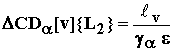7
where DCDa[v] is the difference of Clock Displays (apparent time) on clock aon the moving train during the period when a is traveling across the distance L2. Let us consider clock m. Similarly to clock a, clock m travels during the same time interval, but at velocity v+e. Therefore the DCDµ [v] observed on clock m during the same time interval, will differ only because of the difference of velocity between v and v+e. Since g is velocity dependent, we just have to switch the velocity from ga to gm. The parameter gm, is the value of g corresponding to the velocity v+e of clock m. Similarly to equation 7, the Difference of Clock Display on clock m while clock a travels distance L2 is:8
We have seen that clock b is synchronized with the slow moving clock m, when m reaches b.  After the synchronization of clock b with the arriving clock m, the difference of clock displays between clock a and b (given by clock m), as given by equations 7 and 8 is:9
By definition, we have: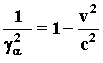10
Since v is very much smaller than c, we can use the series expansion of equation 10.  We get: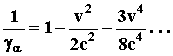11
Since vµ = va+e, we also have: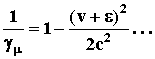12
Equations 11 and 12 in 9 give:13
Using Einstein's synchronization method, equation 13 gives the Difference of Displays, at the same instant, between clocks a and ß. This difference is constant in time. The original Einstein's clock synchronization method was perceived as an attempt to set up an identical display on two remote clocks (a and ß) on the same frame at the same time. However unexpectedly, in a moving frame this synchronization method does not give that expected result (as obtained on the station frame). Equation 13 shows that the Display on clock ß gives an "apparent time" which is earlier than the Display on clock a. This is a fact coming out inevitably from the principle of mass-energy conservation and Einstein's synchronization method. This deficient synchronization of clock ß is responsible for the Sagnac effect that will be explained below. This difference in clock synchronization is normally undetectable and even appears quite natural for an observer traveling inside the moving train.
From the above calculation, we see also that when clock µ returns in the opposite direction (from ß to a), at its arrival, the Clock Display on µ is then again exactly the same as the one carried by the returning clock a. The phenomenon is reversible. We have seen in a previous paper that equation 9.37 which gives the difference between "the difference of clock display on clock ß" minus "the difference of clock display µ" (which has been synchronized with a), is equal to minusvv/c2.  Consequently, since the order of the sub indexes a and ß is reversed, the mathematical sign is changed and equation (13) is identical to equation 9.37.

5 - Table of Clock Synchronization
We have shown above that the synchronization of clocks on a moving frame is such that clocks a and ß must necessarily be synchronized with a different display "at the same instant". This is required even if both clocks a and ß are located on the same frame. However, both clocks (A and B) at each extremity of the station frame show the same display at the same instant. An observer on the station frame could observe that clocks a and ß do not show an identical display at the same instant. However, the observer on the train could not detect any difference when synchronizing his local clocks, because both methods of synchronization using light, or carrying clock µ, agree with the above Einstein's discordant synchronization, between a and ß. Since this phenomenon has not been discussed previously (except in ), and in order to give a non-ambiguous description, we present a table of Clock Displays appearing simultaneously on the four clocks A, B, a and ß as a function of the apparent time on clock A, for each successive second [s] as given in equation 13.

 Clock A  Seconds [s] Clock B  Seconds [s] Clock a Seconds [v] Clock b Seconds [v] 0 0 0 -v/c2 1 1 1/g (1/g)-(v/c2) 2 2 2/g (2/g)-(v/c2) 3 3 3/g (3/g)-(v/c2) ----- ----- ----- -----

Respective Clock Displays on each Clock at the Same Instant.
Table 1

6- Velocity of Light in a Moving Frame
Let us calculate the distance "L2" (see figure 2) traveled by the beam of light emitted at the velocity c, from location A, at rest on the station, during the time light passes from a to ß located in the moving frame.  Using Galilean coordinates, we calculate the velocity of the photons moving at velocity (c-v) with respect to the moving train.  The photons must travel across the moving distance Lv[s] when we consider the relative velocity (c-v) before passing from a to ß.  Consequently, the time TL2[s] (or DCDv[s]) taken to pass from a to ß, at the relative velocity (c-v), is equal to: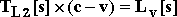14
We havev[s] is the number of rest meters in length Lv[s].  From equation 14 the time for light to travel across L2, can be written: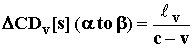15
Multiplying both numerator and denominator on the right hand side of equation 15 by (c+v) and using the definition of g, equation 15 becomes:16
Using equation 4 in 16 we get: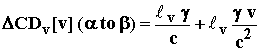17
We have seen above (line after equation 14) that in equation 17 the lengthv is given using rest frame units.  However, the moving observer uses the moving units which is a number g times smaller because the moving standard meter is longer.  Substituting equation 2 in 17, we get: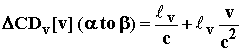18
If we repeat a calculation similar to the equations above, when light is emitted from a source at rest but moving in the opposite direction,  Equations 18 becomes: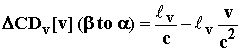19
Equations 18 and 19 show that the time interval for light to travel from a to ß is the sum of two quantities.  The first term (v/c) corresponds to a time interval expected assuming the velocity of light.  The second term must be explained by another phenomenon.
In order to measure the velocity of light in the moving frame, the observer takes the display on clock a when light passes in a. Later when light reaches location ß, he records the display on clock ß.  We have seen in equation 13 that clock ß is late with respect to a.  Consequently the difference of display between clock a and ß after the travel time between the two clocks is given by equation 18 minus equation 13.  This gives:20
When light moves in the opposite direction from ß to a, since clock ß is late with respect to a, we see that equation 13 must be added to equation 19 in order to get the difference of clock display between clock ß and clock a after light traveled between the two locations.  Therefore the difference of clock display between ß and a given by equation 13 plus equation 19 gives: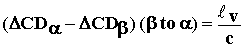21
Equations 20 and 21 explains why the velocity of light appears to be c in the moving frame.  Clocks ß and a are not correctly synchronized as given in equations (20) and (21).  Therefore, constant c in the moving frame is an illusion, because the real velocity is c±v.  The error of synchronization between the two clocks ß and a, as given by equations (20) and (21) compensates exactly, so that the velocity appears to be c, instead of the real c±v that would be measured, if the clocks ß and awould be synchronized correctly.
The error is due to Einstein's clock synchronization method which gives a wrong synchronization.  It is very important to notice that this error in clock synchronization is enormously more important than the usual relativistic correction.  For example, in a frame moving at the velocity of rotation of the Earth, (with (v/c)»0.000001), this correcting term (v v/c2) is one million times larger than the usual correction of g for the change of clock rate used in relativity (which is a function of v2).  It is surprising that this term has not been considered previously, while the relativistic term g, which is much less important (only one part in 1012), is taken into account. This paper deals with this relatively large term (10-6).  A detailed study of the other much smaller (10-12) term will be fully explained later in a future paper.

7 - Experimental Confirmation of the Discordant Einstein's Synchronization Method with the GPS
There are direct measurements proving that the velocity of light in one direction is c±v with respect to the moving observer. This discordant synchronization given in equation 13 has been measured in the world system of clock synchronization with the Global Positioning System. It is then observed experimentally that the Einstein's method of synchronization using the "half time interval" taken by a reflected beam of light is inadequate to determine the correct time. A correction (which is the Sagnac effect) has to be added.
As an example, let us assume that clock a (from figure 2) is in New York (N.Y.), and clock ß is in San Francisco (S.F.) as illustrated on figure 3. The velocity v is the velocity of rotation of the Earth around the pole axis, at the location where the experiment is done. The distanceis the distance between New York and San Francisco (dotted line on figure 3).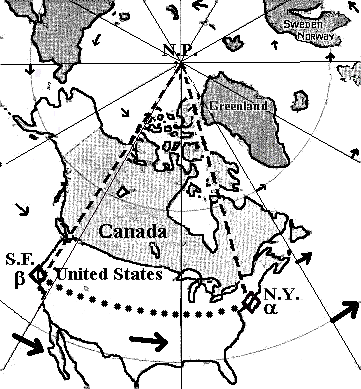Clock Synchronization on the Rotating Earth.
Figure 3

After the initial synchronization of clock a with a mobile atomic clock called µ, that clock is moved from New York to San Francisco at a constant altitude and slow velocity e (see figure 3). The constant altitude (at sea level) avoids other corrections due to the change of gravitational energy, which is irrelevant in this paper. The equivalent of such an experiment has been done by Sadeh  using a truck containing a number of accurate atomic clocks, previously synchronized with a primary standard of time. In the truck, moving clocks were sent down across USA. This experiment is reported in Science . Using the GPS correction (which is mathematically identical to equation 13, the correct time is set up between clock a in New York and clock ß in San Francisco.
The reader must be aware of the fundamental principles of physics involved in the GPS. The standards for the synchronization of clocks stations used by the Global Positioning System have been published in 1990 by the International Radio Consultative Committee: International Telecommunication Union CCIR  which uses similar rules as the 1980 publication of the CCDS (Comité Consultatif pour la définition de la Seconde: Bureau International des Poids et Mesures) .
The Global Positioning System (GPS) determines that after clock µ moves away from clock a in New York, toward clock ß in San Francisco, its display accumulates an extra 14 ns (approximately) with respect to clock ß.  We know that due to the Earth rotation, between N.Y. and S.F. clock µ moves at velocity (v-e), which is the velocity of rotation of the Earth "v" minus the velocity of the truck "e". Therefore 14 ns are subtracted to its display at its arrival in order to give a correct synchronization of time on clock ß in S.F.. This correction is identical to equation 13. This correction is the same as the one programmed automatically in the GPS.
Experimentally, an equivalent experiment has also been done carrying a clock between Washington and Tokyo by Saburi et al. . It is then an experimental fact that the two clocks (a and ß) are not naturally synchronized at the same value, as a result of the discordant Einstein's synchronization method explained above.
There is another well-known way to synchronize the clocks between these two stations (a and ß). It is done sending radio signals transmitted simultaneously (east-west and west-east) between these two cities. Again, it is observed that a simultaneous transmission of radio signals between New York and San Francisco does not give "directly" the same correct clock display (time) in both cities. There is a difference of about 14 ns that must be subtracted to the clock in San Francisco in order to get the correct GPS time. This correction is identical to the one when we are carrying clocks. This correction corresponds to a change of velocity c±v between stations.
This GPS synchronization has been verified in numerous experiments. It is identical to the calculations presented in this paper and also to the Sagnac's effect, (which is included in the GPS). Among the GPS list of corrections, there is a correction involving a parameter taking into account how many Earth meridians are crossed by light or by the moving clock µ, between the two locations. Kelly  explains that the correction used by the GPS is: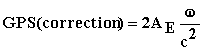22
where w is the angular velocity of rotation of the Earth, AE is the projected area on the Earth equator plane of the path used by light (or by a slowly moving clock) between the two stations. We defineas the distance between the two stations, both moving at velocity v. The circumference of the Earth is called "circ". Therefore the area AE is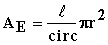23
The angular velocity  w is equal to v/r. The circumference of the Earth is 2pr. Equation 23 in equation 22 gives: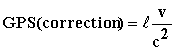24
We see that the GPS correction of clocks (equation 24)  is identical to the Sagnac effect, but also perfectly identical to equation 13. When a clock moves eastward, we understand that the velocity of the clock is added to the Earth velocity so that the term g becomes larger (for the moving mass µ), than for masses a and ß which do not possess that extra velocity. Consequently, the clock moving eastward runs at a slower rate. Consequently, the "Einstein's Clock Synchronization Method" is not compatible with the time given by the GPS and the Sagnac effect must be added. We finally conclude that the difference of clock synchronization given by equation 13 is an experimental fact that has been observed when setting up the Global Positioning System. We must conclude that the velocity of light is equal to c "with respect to the non-rotating frame".

8 - Synchronizing Clocks with the GPS
Other experiments can be realized to test the difference of synchronization (time) between clocks. Experiments, with north-south displacements of clocks, have also been verified experimentally. Instead of exchanging directly the radio signals or moving clocks between New York (N.Y.) and San Francisco (S.F.) as illustrated on figure 3, let us assume that a radio signal is sent from New York to a station at the North Pole (N.P.) of the Earth before being reflected (or re-emitted) toward San Francisco. This can be done using a satellite located above the North Pole. In this case, in agreement with the GPS, we observe that the simultaneous exchange of radio synchronization between a and ß, does not show the difference of 14 ns, since light never travels across meridians, as illustrated on figure 3. Then, light never has to move directly against the Earth velocity of rotation. The projection of the light path on the area A, defined above (equation 23) is zero, because light travels along the meridians, via the North Pole. Of course, there is a higher order correction related to the transverse velocity of light with v that can be considered elsewhere, but this is clearly not observable experimentally.
A similar result is obtained when we carry an atomic clock µ, at constant geodesic altitude in the north-south direction from New York to the North Pole (N.P.). Of course, in that case, clock µ might increase its rate because of the decrease of tangential velocity of Earth rotation at higher latitudes. However, it has been demonstrated that the flatness of the Earth is such that the gravitational potential at the pole compensates exactly for the loss of rotational velocity v. Since no meridians are crossed, the GPS correctly calculates a zero correction on clock µ, at its arrival at the North Pole. For the same reason, a null correction is also calculated on clock µ by the GPS when it is moved from the North Pole (N.P.) to San Francisco (S.F.).
Either using simultaneous light transmission or carrying a clock µ, it is remarkable that both methods of synchronization of clocks between New York and San Francisco, across the North Pole, give an identical zero correction. However, when the radio signal or the moving clock, crosses the meridians, the correction of 14 ns, as calculated by equation 13, appears in both methods.

9 - Observation of the One-Way Velocity of Light as c±v
Knowing that the Sagnac effect, the GPS, all the related experiments described above and also using Newton physics lead to identical results, we can rely on the GPS data. Consequently, the GPS is a reliable tool to measure directly the one-way velocity of light.
Let us start our experiment with an atomic clock at the North Pole of the Earth. At this location, there is evidently no problem with the velocity of Earth rotation (which is non-existent). From the North Pole (N. P.), let us initiate an independent synchronization with the two clocks a and ß located respectively in New York and in San Francisco. Since both methods, (transmission of simultaneous radio signals or carrying an atomic clock), lead to the same result, we can use the synchronization method that we prefer. From the North Pole, and moving along the meridians, the projection of the path on the Earth equator AE is zero. Consequently in that case, synchronizations of the clocks in N.Y. and S.F. with the one at the North Pole, do not need any correction (AE =0 in equation 22).
Therefore, two clocks in San Francisco and in New York are now in perfect synchronization. Using this synchronization, let us measure the velocity of light between N.Y to S.F. and also between S.F. and N.Y. Let the observer in New York send a radio signal (across the meridians) to San Francisco at the same time another radio signal travels in the opposite direction. This simultaneous exchange of radio signals can be done using the refraction of the ionosphere or via a satellite at a low altitude above the same meridian. Since the two clocks have been previously accurately synchronized in the paragraph above, the absolute time of emission and reception can be measured directly on each local clocks (a and ß). If the path length of the radio signal is not much longer than the shortest path (passing across the meridians), the average time interval measured simultaneously in both directions is about 15 000 microseconds.
In that case, an accurate measurement of the time interval given by the synchronization above with the North Pole, (in agreement with the GPS) shows that light takes an extra 0.014 microsecond to travel eastward (from S.F. to N.Y.). Also light arrives at the western station (from N.Y. to S.F.) 0.014 microsecond before the average 15000 microseconds interval needed to travel a distance of about 4500 km. Since there is a difference of 0.014 microsecond between each direction, this shows that light moves at a different velocity eastward than westward. We calculate that the velocity "v" of rotation of the Earth at the latitude of those cities is about one millionth of the velocity of light. From the above data, the time interval for light from New York toward the approaching San Francisco is also about one millionth shorter. Also the time interval for light to move from San Francisco to New York (which has moved away) is about one millionth longer. Clearly, the velocity of light with respect to an observer resting on the Earth surface is c+v between N.Y. and S.F. and c-v between S.F. and N.Y.   One must conclude that the velocity of light is c with respect to a frame at rest.
-
Some people have a restricted interpretation when the say that the velocity of light is c with respect to the non-rotating (zero velocity) frame.   Since the observations above had to be made using the velocity component of the rotating Earth, some people have claimed that the observed change of velocity is directly due to the rotating Earth and consequently, the velocity of light is c "only" with respect to a non-rotating frame.  This naïve conclusion is misleading, because it implies incorrectly that the velocity of light (measured in a moving frame) cannot be different from c when the frame moves in straight line.  We can see that the velocity c±v, measured from a rotating frame, is a special case of velocity c±v due to a linear motion.
-
We can show that logically, the velocity of light is also c±v for an observer moving in straight line (without any rotation).
Let us show first that a statement claiming that light moves at velocity c, “only” with respect to a non-rotating frame is not a physical explanation.  This statement implies that the real velocity of light cannot be c±v, if the frame is not rotating (but just translating).  The word “only” implies that the velocity is c±v in a rotating frame but it would not be c±v in a translating frame (even if the velocity is the same).  There exists no direct physical mechanism capable of explaining that light can move at velocity c±v with respect to a rotating frame and c with respect to a translating frame.  Claiming that the velocity of light is c in a frame translating at velocity v, and simultaneously claiming that the velocity is c±v in a rotating frame, which is the same phenomenon, is incoherent.
In physics, an explanation requires that an observation is coherent with respect to a well-described physical mechanism.  When we claim that the velocity of light is c±v, this is the direct logical consequence of the application of the well-known Galilean coordinates.  With the Galilean coordinates, we understand logically that when a traveler moves in the universe at velocity v with respect to particles moving at velocity c, the velocity of the particles with respect to the moving frame is c-v.  Here the particles are photons.  This last description is a direct physical explanation compatible with conventional logic (without magic).  Therefore the simple statement that, the velocity of light is c, "only" with respect to a non-rotating frame is non-coherent.
-
Let us show now that the velocity of light is c±v with respect to an observer, moving in straight line at velocity v.  Let us consider two flashes of light emitted from our Earth moving in a direction parallel to its motion, during its orbit around the Sun.  The two light beams are emitted simultaneously in opposite directions along the Earth’s orbit.  Around the Sun, there are some stationary stations reflecting light, which are distributed so that light emitted from Earth can circle the Sun simultaneously in both directions.  For example, we can assume eight stationary mirrors reflecting light and forming an octagon around the sun.  For the beam moving in the same direction as the Earth, the moving observer will measure a longer time interval (than in opposite direction) before light completes the rotation around the Sun and reaches Earth again, because the Earth has moved a small distance, during that travel time interval of light.  The velocity of light calculated is then c-v (for the Earth observer).  Also, for the observer on the moving Earth, the velocity will be calculated as c+v for the beam of light moving in the opposite direction.
Between each of the eight pairs of mirrors, in the forward direction, when the observer uses his proper units in his frame and Einstein’s synchronization, the moving observer will “believe” that the velocity of light is c.  This is due to the erroneous clock synchronization explained above.  Also, we could install a GPS around the Sun (as the one on Earth) and we would find, just as on Earth, those clocks are slowing down and standard meters dilated due to the kinetic energy of the observer.  The distance between a pair of mirrors corresponds to the distance between New York and San Francisco on Earth, as in the example above.  Again, the velocity of light moving along a straight line moves at velocity c-v with respect to the observer on Earth, if he uses a correct synchronization method (i.e. from the Sun's pole).
-
The most remarkable thought experiment is when the Sun is completely removed from the center of the system above.  We mean, an experiment done very far in empty outer space.  Then again, the observer moves at velocity v, in straight line, along one side of the octagon.  Due to the observer’s velocity, light will take more time to complete the complete octagonal path when he moves in the same direction as light, than if light and the observer are moving in the opposite direction.  In fact, any mass, either the Earth or the Sun is irrelevant.  Again, this is the classical Sagnac effect.
One must conclude that using traveler’s local units, we always find the same number of local units for the velocity of light, due to the error in Einstein’s clock synchronization demonstrated previously (and also in this paper).  The real velocity of light is really a constant c with respect to an absolute frame at rest.  Consequently, it is (c±v) (and not c) with respect to a moving frame, as measured on a real absolute clock (which takes the variation of units into account), which would not be modified due to its kinetic energy.  The simplest way to make sure that we always use the same time rate, is actually always looking at the "very same clock" (with a telescope if necessary) and correct for the delay of transmission calculated by the observer at rest.
We must conclude that the hypothesis of a constant velocity of light with respect to a moving frame of reference is an illusion and therefore an error.

10 - Absolute Frame of Reference
One must conclude that the GPS and all the related experiments give a striking proof that the velocity of light is not constant with respect to an observer, contrary to Einstein's hypotheses. The measured velocity of light is c-v in one direction and c+v in the other. The velocity of light is equal to c with respect to an absolute frame in space. This is now an experimental fact. Finally, we have seen how it is apparently constant in all frames using proper values and a correct clock synchronization.
We can consider the velocity of light with respect to a group of stars around the Sun. However, there is nothing that says that that star cluster is at an absolute rest. It probably moves around our galaxy which itself moves around the local cluster of galaxies. From what we have seen here, we see that the star cluster mentioned above is just another moving frame, in which again, we have an "apparent" velocity of light equal to c in all directions, because we do not know yet, how to get an absolute synchronization of clocks from the absolute frame.
A simple way does not seem to exist, which would enable us to use light to determine the absolute velocity with respect to the fundamental frame in the universe. We have mentioned in a previous paper  that there seems to be an absolute frame of reference related to the 3K-radiation dipole in space. It exists, however, another solution than the 3K radiation.  Light seems to be inadequate, to verify our absolute velocity with respect to an absolute frame. It exists however another solution to locate that absolute frame, but this is beyond the scope of this paper.
Most physicists believe that the velocity of light is constant with respect to all frames. As explained above, this is wrong.  Let us go back to the question: The velocity of light is "c" with respect to what? The principle of mass-energy conservation requires that light moves at a constant velocity with respect to an absolute frame. Furthermore in all other frames, the velocity of light is always measured to be constant (equal to c) with respect to that moving frame, but it is an "illusion" due to Einstein's discordant clock synchronization.
Some scientists suggest the existence of an "aether" to carry light. A naive "aether" hypothesis leads to a prediction of the velocity of light that could be measured "directly" as c±v with respect to the observer. This is not that simple.  One extremely important point is that there exists no observational justification[10, 11] to assume that an aether can possess its own energy that can be borrowed when needed. On the contrary, all the physical phenomena are explained naturally without having to borrow any energy or momentum from an assumed medium.  For the moment, the sole property of that assumed aether is to establish an absolute origin to the velocity-frame of light and physical matter, because this frame of reference is absolutely needed to comply with the principle of energy and momentum conservation.  That absolute frame might be simply determined by the average velocity of all matter in the universe.
One must conclude that there exists no space-time distortion of any kind. It is no longer necessary to fascinate people with the magic of relativity. Unless we accept the absurd solution that the distance between N.Y. to S.F. is smaller than the distance between S.F. and N.Y., we have to accept that in a moving frame, the velocity of light is different in each direction. As mentioned above, this difference is even programmed in the GPS computer in order to get the correct Global Positioning. This proves that the experimental velocity of light with respect to a moving observer is c±v.

11 - References
 P. Marmet, Einstein's Theory of Relativity versus Classical Mechanics, Newton Physics Books (1997), Gloucester, Ontario, Canada K1J 7N4.  Book on the Web.
 P. Marmet, Classical Description of the Advance of the Perihelion of Mercury, Physics Essays Vol. 12. No: 4, 1999. Web page
 Sadeh et al. Science 162, 897-8 (1968)
 Straumann N. General Relativity and Relativistic Astrophysics, Springer-Verlag, Berlin, Second printing 1991, pp. 459,
 CCIR Internat. Telecom, Union Annex to Vol 7, No: 439-5. Geneva 150-4 (1990).
 CCDS Bur. Int. Poids et Mes. 9th Sess., 14-17, 1980.
 Saburi et al. IEEE Trans. IM25, 473-7, 1976.
 A. G. Kelly, "The Sagnac Effect and the GPS Synchronization of Clock-Stations" Ph. D. HDS Energy Ltd., Celbridge, Co. Kildare, Ireland. Also, A. G. Kelly, Inst. Engrs. Ireland 1995 and 1996, Monographs 1 & 2.
 P. Marmet, The Origin of the 3K Radiation, Apeiron Vol, 2 Nr, 1. January 1995 Web page
 P. Marmet, Classical Description of the Advance of the Perihelion of Mercury, Physics Essays, Vol. 12, No: 3, 1999.
 P. Marmet, C. Couture, Relativistic Deflectionof Light Near the Sun Using Radio Signals and Visible Light, Physics Essays, Vol. 12, No: 1 (1999) Web page

---

<><><><><><><><><><><><>

Return to:    List of Papers on Web Sites

<><><><><><><><><><><><>
A similar paper: "Explaining the Illusion of the Constant Velocity of Light" has been presented at the Meeting "Physical Interpretations of Relativity Theory VII" University of Sunderland, London U.K., 15-18, September 2000. This report appears in the Conference Proceedings "Physical Interpretations of Relativity Theory VII" p. 250-260 (Ed. M. C. Duffy, University of Sunderland).
---

The same ideas as below also appears in the Magazine "Acta Scientiarum" (2000) under the title: "The GPS and the Constant Velocity of Light"

============

IMPORTANT NOTE:
Another paper related to the one here above, has been published under the title  "GPS and the Illusion of Constant Light Speed" in Galilean Electrodynamics", Vol. 14, No:2,  p. 23-30.  March/April 2003.  Unfortunately, a word has been mistakenly added, which makes the sentence totally erroneous.  That sentence is:  "The speed of light is c ONLY with respect to a non-rotating frame".  The word ONLY did not exist in the original author’s paper.
Unfortunately, that sentence implies that the velocity of light is necessarily c when a frame does not rotate.  That is an error, as demonstrated here above in section 9.

P.M.
<><><><><><><><><><><><>

Revised - Nov. 2000